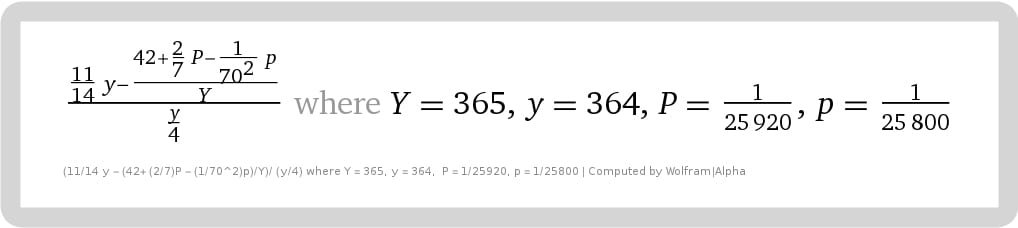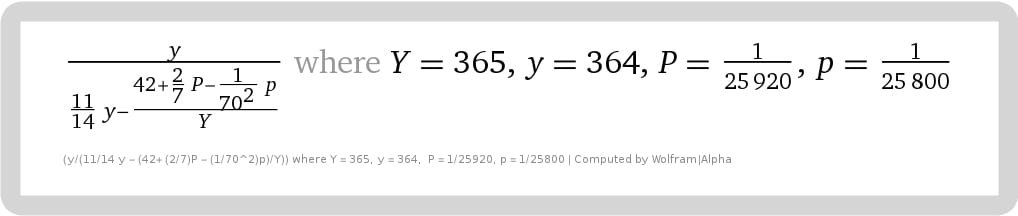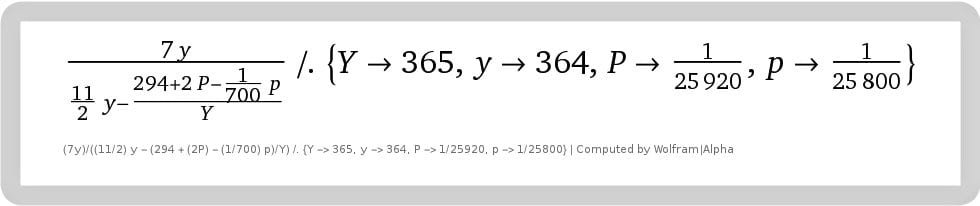## Enoch’s rod of π is the revelation of Jesus

(This represents my earliest work on Pi. While accurate, the final formula is explained at this link.)

The following formula is 1000 times more accurate than NASA uses for all its space endeavors. Yet it unfolds Enoch’s calendar (and all calendars) and is the numerical sum of much that is found in nature and the Bible.

As discussed in previous documents, (written before this formula was discovered)…

• 364 is a year on Enoch’s calendar
• 365 is a solar Year
• 25920 and 25800 years are the “Great Year“, that is, Precession of the Equinox. And as the 1/25920th part of a day (and thus 1/25800th, too), it is the smallest unit according to Babylonian and Rabinnic reckoning (= 3.33… seconds)Pi: The Rod of Enoch. This formula locates a position in space/time of a straight line surrounded by its circle, which by definition is πPi: The Rod of Enoch. The formula locates a position in space/time of the perimeter of the square to its corresponding circle, symbolic of earth and water respectivelyPi: The Rod of Enoch, here converted into units of seven (“weeks”) instead of “one” that reveal other relationships to the Enoch calendar. (Example, “the week” and “294” (7*7*6) are crucial cycles in the calendar. Moreover, keep in mind that a day is as a year (or “a 1000 years”) and a year is as a day”, (2Peter 3:8)

Below is the formula of the ratio of Perimeter to Circumference expressed as a fraction.

226748176200000/178087601141201

y/((11/14) y – (42 + (2/7) P – (1/70^2) p)/Y) /. {Y -> 365, y -> 364, P -> 1/25920, p -> 1/25800}

(Four divided into the above = π rationalized, i.e., 178087601141201/56687044050000.)

### The formula multiplied by 1 season of Enoch (91)An expanded version of the formula for Pi in space and time. Keep in mind that “1274” is simply 364*3.5, and is, therefore, the equivalent of 1260 days (Rev. 11). The 1000 years begins the last Sabbatical cycle of seven-year periods that “cuts short” 21 weeks, less “the twinkling of an eye” of the full 1001 years. In part, it has to do with the tithe (10%). Example, 441 + 49 = 490 (Dan 9); 7*365 less 10% = 2300 (Dan 5,8,12), and here, +/- 10% of 1000 (and 1oo1) x 365 (0r 360 or 364) (&*4), etc.

I will elaborate later, especially the rationalization of π using continual fractions, how that it unravels the above formula in stages.

## The Math of the Bible Fractals

It is also the math of the fractals and its arrangements.